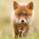1033 views
1033
Request for JR, added fast MA's and conditions, modified the sl lines to display as lines and added option to show/hide them, modified shape to display arrows up/down
study(title="[RS]JR Moving Average System V1", shorttitle="[RS]JR.MAS.V1", overlay=true)
hide_sl_lines = input(true)
ma0 = sma(hl2, input(1))

tp = input(8)
ma1 = wma(open, tp)
ma2 = ema(open, tp)

//---   2nd pass
tf = input(2)
ma3 = wma(open, tf)
ma4 = ema(open, tf)
//---   2nd pass plot output not required.

plot(ma0, color=black)
plot(ma1, color=gray)
plot(ma2, color=silver)

condition = ma0 >= ma1 and ma1 >= ma2 ? (close >= open ? lime : green) :
ma0 <= ma1 and ma1 <= ma2 ? (close >= open ? red : maroon) :
ma0 >= ma3 and ma3 >= ma4 ? (close >= open ? lime : green) :
ma0 <= ma3 and ma3 <= ma4 ? (close >= open ? red : maroon) : (close >= open ? silver : gray)

barcolor(condition)

//  ||---   Crossovers
buyx1 = cross(ma0,ma1) and ma0 >= ma0 ? true : false
sellx1 = cross(ma0,ma1) and ma0 <= ma0 ? true : false
plotshape(sellx1, style=shape.arrowdown, color=maroon, location=location.abovebar)

//  ||---   SL lines
sltop = highest(max(max(high,ma0),max(ma1,ma2)), tp)
slbot = lowest(min(min(low,ma0),min(ma1,ma2)), tp)

tcol = sltop != sltop ? na : silver
bcol = slbot != slbot ? na : silver
plot(hide_sl_lines ? na : sltop, color=tcol, style=line)
plot(hide_sl_lines ? na : slbot, color=bcol, style=line)When rules are applied 2,8,2,8 Ichimoku gave no false signals for the chart above.QuantitativeExhaustion
for ex., input(2.0, title="Mult") will allow user to enter decimal values for conversion line, lagging line and span/displacement.QuantitativeExhaustion
opening above / under the cloud isnt enought as a filter?
it would filter theseRicardoSantos
Are you sure?

looking around March 6th I see a negative lead. I don't see how we could have gotten green candles.RicardoSantos
Is that for the two Ichimoku signals alone? I'd wait on adding any other indications with Ichimoku.x=input("240", title="Enter mins")

Above was mentioned by LazyBear as a way to include MTF inputs by the user for anytime frame.